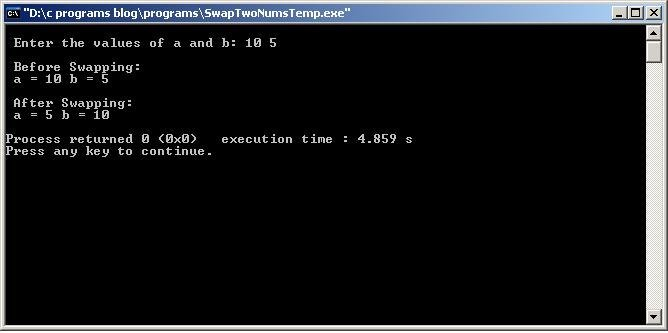# C Program to Swap Two Numbers using Temp/Third Variable

/* C Program to Swap Two Numbers using Temp/Third Variable */

```#include <stdio.h>
main()
{
int a, b, temp;

printf("\n Enter the values of a and b: ");
scanf("%d %d", &a, &b);  // Taking The values of x and y from user
printf("\n Before Swapping: \n a = %d b = %d \n",a,b);

temp = a; //temp stores value of a
a = b;    //a stores b value
b = temp; //b stores temp value

printf("\n After Swapping: n a = %d b = %d \n",a,b);
return 0;
}```
Output: ( using GNU GCC Compiler with Code Blocks IDE )